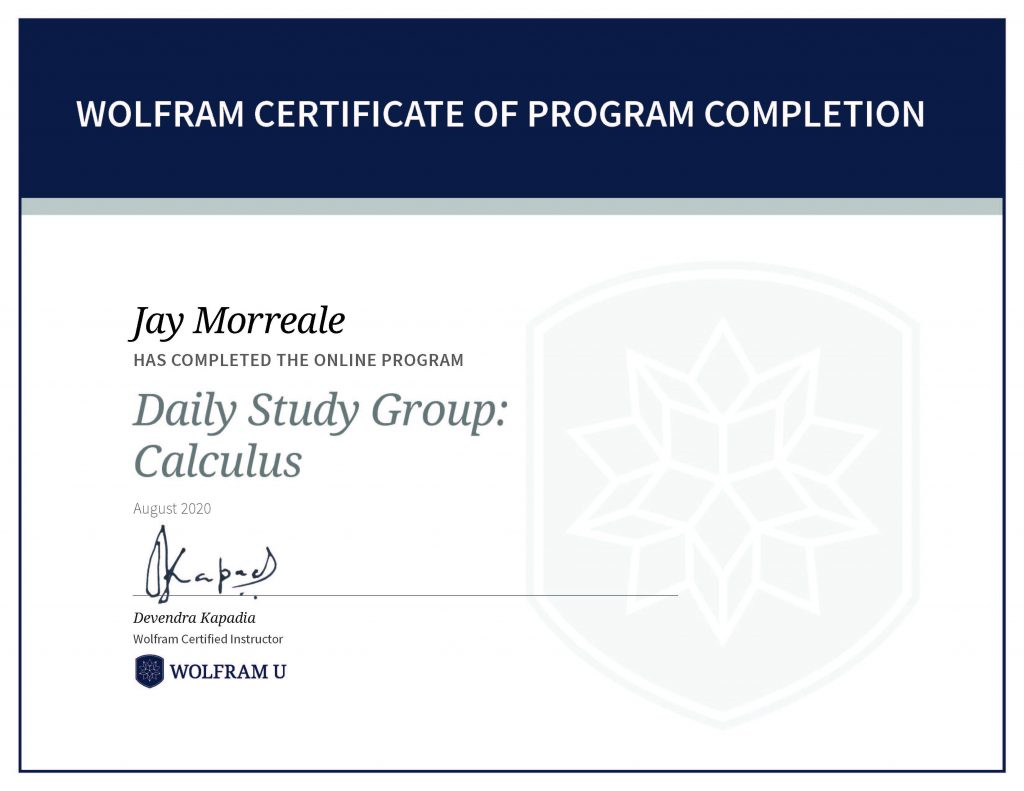# Wolfram U Daily Study Group: Analyzing COVID-19 Data

The week long Analyzing COVID-19 Data Study Group (6) covered the of analysis, visualization and modeling of COVID-19 pandemic data using various Wolfram COVID-19 resources, the NY Times COVID-19 Dataset, and a patient data set. The daily study sessions demonstrated techniques to analyze patient data, virus genetic sequences, and model the pandemic using epidemic data. The figure below shows the COVID-19 Death Rate per 100,000 people by state based on the April 11, 2021 update of the Epidemic Data for Novel Coronavirus COVID-19 dataset, for example.COVID-19 Death Rate per 100,000 people by state using the April 11, 2021 Wolfram dataset.Wolfram Certificate of Program Completion, Jay Morreale has completed the online program Daily Study Group: Analyzing COVID-19 Data.

# Wolfram U Daily Study Group: System Modeling

The two week long System Modeling Study Group (5) uses the Wolfram System Modeler: An Introduction course as part of the day lectures. The Wolfram System Modeler is a separate application and is based on the open source Modelica language. In the study group, we simulated chemical reactions, thermal conductivity of walls, the electro-mechanics of a joystick, a DC motor, and a heater, for example. The System Modeler uses the Functional Mock-up Interface (FMI) and can interface with more that 150 tools. We installed StandardFirmata into an Arduino to demonstrate a control applications with System Modeler.Wolfram Certificate of Program Completion, Jay Morreale has completed the online program Daily Study Group: System Modeling.

# Wolfram U Daily Study Group: Calculus

The three week Calculus Study Group (4) uses the Introduction to Calculus self study course as part of the daily lectures and covers functions, limits, differential calculus, and integral calculus. This course is a good way to prepare for AP Calculus and is a great refresher on the topic.

The functions 3x, the derivative of 3x, ex, the derivative of ex, and 2x, the derivative of 2x are shown in the plot on the left in the figure below to show the awesome properties of ex, for example. The derivative of ex is ex so the slope anywhere on the line is just the value of the function at that point.

The tangent line and normal line are shown in the plot on the right for ex=3. This point was chosen as an example. The functions used to create the tangent and normal lines are as follows.

• f(x)=ex
• Tangent(x)=f′(x)(x−x0)+f(x0) at x0=3
• Normal(x)=(−1/f′(x))(x−x0)+f(x0) at x0=3

The area under ex to x0=3 can be found by integrating the curve from −∞ to 3 and is e3. Thus, the area under the curve is also the value of the function at that point.

To receive a program completion certificate you must pass three quizzes.Wolfram Certificate of Program Completion, Jay Morreale has completed the online program Daily Study Group: Calculus.

# Wolfram U Introduction to Notebooks

The three week Introduction to Notebooks Study Group (3) covered notebooks, machine learning, and image processing. Daily study group lectures included content from Wolfram courses and live instructor instruction that varied from week to week and included:

• Week 1: Introduction to Notebooks covered styling, formatting and creating interactive documents.
• Week 2: Machine Learning covered classification, regression, cluster analysis and anomaly detection.
• Week 3: Image processing covered digital image processing, image representation, and classical operations on images.

I used the image processing tools to find clusters in a photograph of an Arduino MKR WiFi 1010 board, colorized the clusters, and add colorized text to make a composite illustration that demonstrates the use of these features.